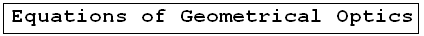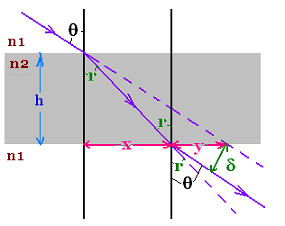Geometrical Optics: Geometrical Optics & Physics Optics. a Huygens Principle: Wavefronts. a Reflection: The first law of Geometrical Optics. a Refraction: The second law of Geometrical Optics: Snell's law. a Critical angle: Internal total reflection. a images formed by reflection Spherical concave mirrors. Mirror equation. a images formed by refraction Spherical convex mirrors .. a Fermat's principle: Fermat's principle for reflection and refraction.. a lenses: Thin lenses and Lens-makers' equation .. a prism: Prism, colors minimum deviation .. a Human eye: near point, far point, nearsightedness, farsightedness.. a Microscope: Microscope: simple, compound, and magnification.. a Telescope: Telescope: magnification.. a Resolution: optical microscope, electron microscope .. a dispersion: dispersion, more about the refractive index, and colors of a prism .. a parallel sheet: deviation by a parallel sheet .. a thin films : thin films and Newton's rings.. a Newton's rings: thin films and Newton's rings.. a Descartes rainbow: Primary and secondary rainbows .. a Some applications More fun with Optics .. a Optics calculator: Optics calculator: all the related calculations .. a home The fundamental, and just this .. a scientificSentence a __________________ a

Geometrical Optics
Optics -     Geometrical Optics..

### Deviation by a parallel sheetA light ray is refracted at the surface (interface) n1-n2, crosses the parallel sheet of thickness "h", and emerges at the surface n2-n1. The incidence angle is θ. we are interested to know the deviation δ.

First, the ray emerges parallel to the incident ray. Indeed, according to Snell's law, for the interface n1-n2, n1 sin θ = n2 sin r; and for the interface n2-n1, n2 sin r = n1 sin (angle of emergence). Therefore, angle of emergence = θ and the incidence ray is parallel to the emerged ray.

We have:
x = h tan r
x + y = h tan θ
Then:
y = h tan θ - x = h tan θ - h tan r
Substituting in this relationship the expression of sin r taken from Snell's law, n1 sin θ n2 sin r → sin r = (n1/n2) sin θ, we obtain:

y = h tan θ - x = h tan θ - h sin r/ cos r
= h tan θ - h sin r/ (1 - sin2r)1/2
= h tan θ - h (n1/n2) sin θ)/ (1 - [(n1/n2) sin θ]2)1/2
= h tan θ [1 - (n1/n2) cos θ/ (1 - [(n1/n2)2 sin2 θ])1/2]

y = h tan θ [1 - (n1/n2) cos θ/ (1 - [(n1/n2)2 sin2 θ])1/2]

We have also: δ = y cos θ. Then, finally,
δ = h sin θ [1 - (n1/n2) cos θ/ (1 - [(n1/n2)2 sin2 θ])1/2]

δ = h sin θ [1 - (n1/n2) cos θ/ (1 - [(n1/n2)2 sin2 θ])1/2]

 @import url(http://www.google.com/cse/api/branding.css);Custom Search © 2009. The scientificsentence . All rights reserved.